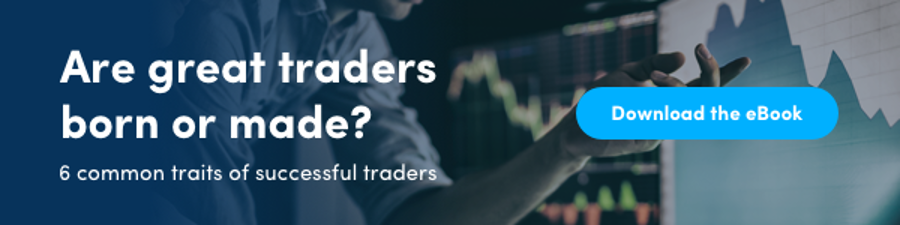# How do good traders use leverage? (Part II)

This post continues the discussion on optimal leverage that we began in the first part of this series.

Today we shed light on the hypothesis, modelling and results phases of a study conducted by Darwinex Labs, wherein they quantitatively demonstrated the long-term impact of incremental leverage on trading strategy performance.

Particular emphasis was laid on simulating a trader’s choice of leverage and the associated likelihood of successful recovery in the advent of a loss.

By the end of this post, traders will hopefully develop a better understanding of how leverage affects their chances of survival in the financial markets and be better equipped to decide what leverage they consider most optimal, depending on their risk appetite.

Before delving into the details of how we conducted this research, let’s first simplify the concept of Value-at-Risk (VaR) a little for the benefit of all our readers.## Demystifying Value-at-Risk (VaR)

VaR is based on the probability distribution of returns from an asset, portfolio and/or trading strategy (DARWIN).

One of the main premises behind the concept is that all liquid financial instruments exhibit:

• Varying degrees of uncertainty
• .. due to varying degrees of market risk
• …. from various sources of risk, depending on the instrument(s) in question.

It’s this uncertainty that can be modeled with appropriately chosen probability distributions.

To keep things straightforward for the purpose of this post, bearing the following two concepts in mind will serve you well.

Simply put, Value-at-Risk is a percentile of loss, derived from:

1. A model – the part used to calculate the VaR.
2. A measure – what we infer from the output of the model.

The model is used to calculate a return accompanied by a probability of the asset or portfolio losing more than this return over a given time horizon. i.e. it is a statistical measure of the losses possible when taking on market exposure.

### For example,

A VaR model applied to a trading strategy may output “Monthly VaR (95%) = -21.0%”, which translates to “there is a 5% chance of this trading strategy losing more than -21.0% in any given month”.With that in mind, let’s now discuss the specifics of the study itself.

## Question

Beyond what VaR does loss recovery become statistically improbable?

The answer to this question isn’t at all straightforward to decipher quantitatively.

Indeed as you’ll see shortly, though our findings gave us conclusive evidence that using excess leverage is not in the best interests of a trader, we weren’t able to pinpoint an exact or tight range to answer this question satisfactorily.

We therefore leave it up to readers to form their own conclusions based on this study’s findings.

## The Model

To calculate the VaR and other descriptive statistics, we needed to construct a probability distribution of returns for each level of leverage we wanted to test.

To generate these returns, we first created the following trading strategy:

EUR/USD

Direction:

Execute 1 trade per day, lasting exactly 1 hour, for 1 month, with randomly chosen directions and starting times.

Simulations:
100,000 (repeat trading logic this many times)

Leverage:
1:1 to 400:1 (repeat trading logic for each leverage level in this range, in steps of 10)

Logic dictates that the higher the leverage used for trading, the greater the risk of not being able to recover the account in the advent of a large loss, as a result of running out of the capital required to maintain that leverage assuming capital in the account is the trader’s only available capital.

Running the algorithm above produced the following results, attesting to the validity of this assumption:

Chart 1: Probability Density Distribution of Returns by Leverage (click to enlarge)Observations:

As leverage increases, all 3 of the following metrics worsen considerably:

1. Monthly VaR (95% confidence)
2. Median Return
3. Probability of recovering loss of median return – P(Recovery)

This behaviour presents itself in the chart above, in the form of systematic shifting to the left of each distribution with higher leverage than the prior.

Chart 2: Evolution of Median Return vs. Leverage (click to enlarge)Observations:

After an initial, briefly slow rate of change, median return degradation accelerates to an optimum, systematically worsening as leverage increases.

Chart 3: Evolution of VaR (95% confidence) vs. Leverage (click to enlarge)Observations:

Past a certain choice of leverage, VaR converges to -100%, ruling out high leverage as a means nor solution to the “trade peanuts, make peanuts” problem discussed in Part I of this series.

Chart 4: Probability of Median Recovery vs. Leverage (click to enlarge)Observations:

As the leverage increases, the probability of recovering a loss of median return in the next month, systematically decreases.

## Conclusions

The findings of this study further reinforce some of the assertions made earlier in this post, as well as conclusions made in Part I of this series:

1. Trading beyond a certain VaR makes it statistically improbable that the trader will be able to recover a loss of median return in the next month.
2. It makes more sense for DARWIN providers to employ stable, moderate to low VaR and leverage investor capital instead.
3. Traders stand to benefit from analyzing their trading strategies in this manner, in order to take preemptive action should their strategies be at risk of statistically irrecoverable loss.Tags:
1.